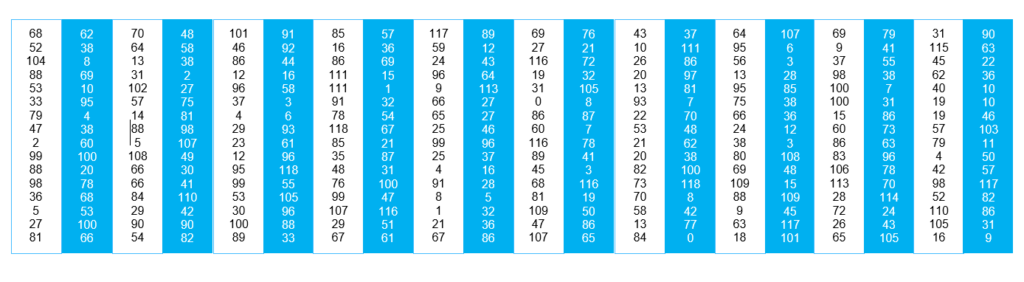# Logic and/or IQ-table.

Logical-political tips from Rodenskolan, Norrtälje 1987:

(SSU 1)

One can read conclusions directly from the logic table with ruler and Latin intersection. 1+2=3

(SSU 2)

There can be logical decimal numbers from little to much like the camels’ humps. Attendance at school can sometimes be less than 100% but greater than 0.

binary numbers 0; 1 continuous decimal numbers 0; 0.46721 0.98 1

(MUF 1) 0<1

The 5 most common logic functions and the computers NAND, NOR, XOR can be generalized to 16 basic functions in the logic table. If you have blue stripes in the logic table, it looks like a metaphor of the Greek flag.

(MUF 2)

Even statements with negation can be true. Ex: The color of the house is not sky blue.

(Mathematics and logics1) π = 3,141592654…

The logical 16 + 2 basic functions can be assembled into new poly-logical functions such as Blocking, Necesse, the ”Twins paradox”, Nirvana, etc. The number of logical functions can go towards infinity infinitum and the yellow logical color increases; one does not always know certain conclusions.

(Mathematics education2) Trigonometry with sine function can go from 0 to 1 continuously, so many decimal numbers exist between extreme values 0 to 1. Ex: 50% 0,5 0,79813246 0,19789851334842121 …

Examples of extended logic: ”2=1” All as definition can be number 2 among all arabic numbers, and for a set be all 100%. Thus 2 can be egual to 1 and true. (?)

0=0 If there is equivalence that is 1=1 but zero must also be reminded. A customer just have a look and second customer also takes just a look, thus 0=0! A customer that not buys articles do not have to pay. (Line in logic table) (?)IQ-table where the subquantity IQ derives from the English language. The abbreviation IQ means intelligence quota.

IQ-table in RGB-colors or RGBY-colors where Y means yellow. The colors are an approximation system system according to the rainbow where all colors exist. The free definition under this system is:

RED top IQ

YELLOW (fr. 0U1?) you may not know other people’s IQ?

GREEN average (fr. moyen) si-sådär comme-ci comme ça ”medelmåtta” ”normalbegåvad”

BLUE moderate talent